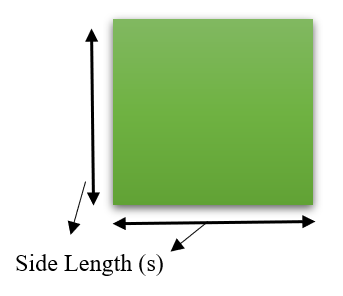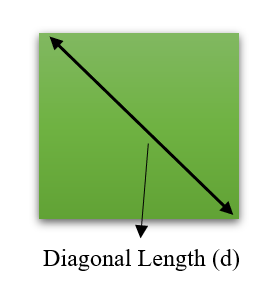# Area of a Square Formula

• Last Updated : 28 Jan, 2022

Square is a Two-Dimensional (2D) figure which has 4 sides of all equal length. The area of a square concept comes under the topic mensuration which deals with the measurements of Two Dimensional and Three Dimensional figures. Length, Perimeter, Area, Volume etc. come under measurements of a figure.

### Area of Square

The area is the region inside the boundaries of an object i.e., square. The area of a square is defined as the number of square units needed to fill the square. To calculate the square area we need to know the length of any side. The area of the square can be calculated by finding the square of the side length.### Area of Square Formula using Sides

Area of Square = Side2

Let’s look at the few examples to get more understanding on this.

Question 1: What is the area of a square if each side of length is 4cm.

Solution:

Given

Side length (s) = 4cm

Area of square = s2

= 42

= 16cm2

The area of square with side length 4cm is 16cm2.

Question 2: The length of each side of a square is 5cm and the cost of painting it is Rs. 5 per sq. cm. Find the total cost to paint the square.

Solution:

Given

Side length (s) = 5cm

Area of square = s2

= 52

= 25cm2

For 1 sq.cm cost of painting is Rs 5.

Total Cost of painting the 25sq cm= 25 × 5 = Rs125

There is an alternate way to find the area when the diagonal length of a square is given

### Area of Square Formula (Diagonal Given)Area of Square when diagonal length is given,

Area = (1/2) × d2

Where d is the length of diagonal.

Let’s look into an example

Question: Find the area of a square if the length of the diagonal is 6cm.

Solution:

Given

Diagonal length (d) = 6cm

Area = (1/2) × d2

= (1/2) × 62

= 36/2

= 18cm2

Area of square is 18cm2.

### Calculating Area of the square from Perimeter

We can find an area even from the perimeter is given for a square.

The perimeter formula for a square = 4 × side

From the above formula, we can find side length by dividing Perimeter by 4.

So, Side length = Perimeter/4

Using side length we can find the area of the square by calculating the square of a side.

Let’s solve a few examples of it.

Question 1: What is the Area of a Square if the perimeter of a square is 24cm.

Solution:

Given

Perimeter = 24cm

So, Side length = perimeter/4

Side(s) = 24/4

= 6cm

From the side length we can calculate area of square by

Area = Side2

= 62

= 36cm2

The area of square with given perimeter is 36cm2.

Question 2: Find the Area of Square if the perimeter of a square is 64cm.

Solution:

Given

Perimeter=64cm

So, Side length=perimeter/4

Side(s) = 64/4

= 16cm

From the side length we can calculate area of square by

Area=Side2

= 162

=256cm2

The area of square with given perimeter is 256cm2.

These all are the possible ways to find the area of the square.

My Personal Notes arrow_drop_up